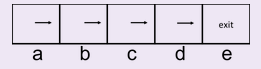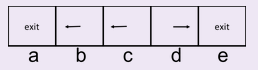# Quiz: Markov Decision Process¶

Is the following statement True or False?

If the only difference between two MDPs is the value of the discount factor then they must have the same optimal policy.

Is the following statement True or False?

For an infinite horizon MDP with a finite number of states and actions and with a discount factor γ that satisfies 0 < γ < 1, value iteration is guaranteed to converge.

Is the following statement True or False?

When running value iteration, if the policy (the greedy policy with respect to the values) has converged, the values must have converged as well.

Is the following statement True or False?

If one is using value iteration and the values have converged, the policy must have converged as well.

Is the following statement True or False?

For an infinite horizon MDP with a finite number of states and actions and with a discount factor γ that satisfies 0 < γ < 1, policy iteration is guaranteed to converge.

Is the following statement True or False?

“Q-values” are determined by immediate expected reward plus the best utility from the next state onwards.Note: One round of policy iteration = performing policy evaluation followed by performing policy improvement.

Is the following statement True or False?

It is guaranteed that ∀s ∈ S : V π James (s) ≥ V π Alvin (s)Note: One round of policy iteration = performing policy evaluation followed by performing policy improvement.

Is the following statement True or False?

It is guaranteed that ∀s ∈ S : V π Michael (s) ≥ V π Alvin (s)Note: One round of policy iteration = performing policy evaluation followed by performing policy improvement.

Is the following statement True or False?

It is guaranteed that ∀s ∈ S : V π Michael (s) > V π John (s)Note: One round of policy iteration = performing policy evaluation followed by performing policy improvement.

Is the following statement True or False?

It is guaranteed that ∀s ∈ S : V π James (s) > V π John (s)

Is the following statement about value iteration True or False? We assume the MDP has a finite number of actions and states, and that the discount factor satisfies 0 < γ < 1.

Value iteration is guaranteed to converge.

Is the following statement about value iteration True or False? We assume the MDP has a finite number of actions and states, and that the discount factor satisfies 0 < γ < 1.

Value iteration will converge to the same vector values (V*) no matter what values we use to initialize V.

What is the value of the discount factor γ if we want to maximize immediate rewards?
In an MDP with consisting of 2 states and finite action space consisting of 4 actions, what is the dimension of the transition probability matrix? (multiply all dimensions together)Consider the gridworld MDP for which Left and Right actions are always successful. Specifically, the available actions in each state are to move to the neighboring grid squares. From state a, there is also an exit action available, which results in going to the terminal state and collecting a reward of 10. Similarly, in state e, the reward for the exit action is 1. Exit actions are always successful. Immediate rewards at other squares are 0. Let the discount factor γ = 0.2. Fill in the following quantity. V*(a) = V (a) =Consider the gridworld MDP for which Left and Right actions are always successful. Specifically, the available actions in each state are to move to the neighboring grid squares. From state a, there is also an exit action available, which results in going to the terminal state and collecting a reward of 10. Similarly, in state e, the reward for the exit action is 1. Exit actions are always successful. Immediate rewards at other squares are 0. Let the discount factor γ = 0.2. Fill in the following quantity. V*(b) = V (b) =Consider the gridworld MDP for which Left and Right actions are always successful. Specifically, the available actions in each state are to move to the neighboring grid squares. From state a, there is also an exit action available, which results in going to the terminal state and collecting a reward of 10. Similarly, in state e, the reward for the exit action is 1. Exit actions are always successful. Immediate rewards at other squares are 0. Let the discount factor γ = 0.2. Fill in the following quantity. V*(c) = V (c) =Consider the gridworld MDP for which Left and Right actions are always successful. Specifically, the available actions in each state are to move to the neighboring grid squares. From state a, there is also an exit action available, which results in going to the terminal state and collecting a reward of 10. Similarly, in state e, the reward for the exit action is 1. Exit actions are always successful. Immediate rewards at other squares are 0. Let the discount factor γ = 0.2. Fill in the following quantity. V*(d) = V (d) =Consider the gridworld MDP for which Left and Right actions are always successful. Specifically, the available actions in each state are to move to the neighboring grid squares. From state a, there is also an exit action available, which results in going to the terminal state and collecting a reward of 10. Similarly, in state e, the reward for the exit action is 1. Exit actions are always successful. Immediate rewards at other squares are 0. Let the discount factor γ = 0.2. Fill in the following quantity. V*(e) = V (e) =Consider the gridworld where Left and Right actions are always successful. Specifically, the available actions in each state are to move to the neighboring grid squares. From state a, there is also an exit action available, which results in going to the terminal state and collecting a reward of 10. Similarly, in state e, the reward for the exit action is 1. Exit actions are always successful. The discount factor γ = 1. Consider the policy π shown below, and evaluate the following quantity for this policy.V π (a) =Consider the gridworld where Left and Right actions are always successful. Specifically, the available actions in each state are to move to the neighboring grid squares. From state a, there is also an exit action available, which results in going to the terminal state and collecting a reward of 10. Similarly, in state e, the reward for the exit action is 1. Exit actions are always successful. The discount factor γ = 1. Consider the policy π shown below, and evaluate the following quantity for this policy.V π (b) =Consider the gridworld where Left and Right actions are always successful. Specifically, the available actions in each state are to move to the neighboring grid squares. From state a, there is also an exit action available, which results in going to the terminal state and collecting a reward of 10. Similarly, in state e, the reward for the exit action is 1. Exit actions are always successful. The discount factor γ = 1. Consider the policy π shown below, and evaluate the following quantity for this policy.V π (c) =Consider the gridworld where Left and Right actions are always successful. Specifically, the available actions in each state are to move to the neighboring grid squares. From state a, there is also an exit action available, which results in going to the terminal state and collecting a reward of 10. Similarly, in state e, the reward for the exit action is 1. Exit actions are always successful. The discount factor γ = 1. Consider the policy π shown below, and evaluate the following quantity for this policy.V π (d) =Consider the gridworld where Left and Right actions are always successful. Specifically, the available actions in each state are to move to the neighboring grid squares. From state a, there is also an exit action available, which results in going to the terminal state and collecting a reward of 10. Similarly, in state e, the reward for the exit action is 1. Exit actions are always successful. The discount factor γ = 1. Consider the policy π shown below, and evaluate the following quantity for this policy.V π (e) =Consider the gridworld where Left and Right actions are always successful. Specifically, the available actions in each state are to move to the neighboring grid squares. From state a, there is also an exit action available, which results in going to the terminal state and collecting a reward of 10. Similarly, in state e, the reward for the exit action is 1. Exit actions are always successful. The discount factor γ = 1. Now, consider the policy π shown below, and evaluate the following quantity for this policy.V π (a) =Consider the gridworld where Left and Right actions are always successful. Specifically, the available actions in each state are to move to the neighboring grid squares. From state a, there is also an exit action available, which results in going to the terminal state and collecting a reward of 10. Similarly, in state e, the reward for the exit action is 1. Exit actions are always successful. The discount factor γ = 1. Now, consider the policy π shown below, and evaluate the following quantity for this policy.V π (b) =Consider the gridworld where Left and Right actions are always successful. Specifically, the available actions in each state are to move to the neighboring grid squares. From state a, there is also an exit action available, which results in going to the terminal state and collecting a reward of 10. Similarly, in state e, the reward for the exit action is 1. Exit actions are always successful. The discount factor γ = 1. Now, consider the policy π shown below, and evaluate the following quantity for this policy.V π (c) =Consider the gridworld where Left and Right actions are always successful. Specifically, the available actions in each state are to move to the neighboring grid squares. From state a, there is also an exit action available, which results in going to the terminal state and collecting a reward of 10. Similarly, in state e, the reward for the exit action is 1. Exit actions are always successful. The discount factor γ = 1. Now, consider the policy π shown below, and evaluate the following quantity for this policy.V π (d) =Consider the gridworld where Left and Right actions are always successful. Specifically, the available actions in each state are to move to the neighboring grid squares. From state a, there is also an exit action available, which results in going to the terminal state and collecting a reward of 10. Similarly, in state e, the reward for the exit action is 1. Exit actions are always successful. The discount factor γ = 1. Now, consider the policy π shown below, and evaluate the following quantity for this policy.V π (e) =

Posting submission...